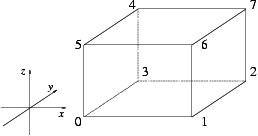CGAL 4.7 - 2D and 3D Linear Geometry Kernel
CGAL::Iso_cuboid_3< Kernel > Class Template Reference

#include <CGAL/Iso_cuboid_3.h>

## Definition

An object c of the data type Iso_cuboid_3 is a cuboid in the Euclidean space $$\E^3$$ with edges parallel to the $$x$$, $$y$$ and $$z$$ axis of the coordinate system.

Although they are represented in a canonical form by only two vertices, namely the lexicographically smallest and largest vertex with respect to Cartesian $$xyz$$ coordinates, we provide functions for "accessing" the other vertices as well.

Iso-oriented cuboids and bounding boxes are quite similar. The difference however is that bounding boxes have always double coordinates, whereas the coordinate type of an iso-oriented cuboid is chosen by the user.

Kernel::IsoCuboid_3

## Creation

Iso_cuboid_3 (const Point_3< Kernel > &p, const Point_3< Kernel > &q)
introduces an iso-oriented cuboid c with diagonal opposite vertices p and q. More...

Iso_cuboid_3 (const Point_3< Kernel > &p, const Point_3< Kernel > &q, int)
introduces an iso-oriented cuboid c with diagonal opposite vertices p and q. More...

Iso_cuboid_3 (const Point_3< Kernel > &left, const Point_3< Kernel > &right, const Point_3< Kernel > &bottom, const Point_3< Kernel > &top, const Point_3< Kernel > &far, const Point_3< Kernel > &close)
introduces an iso-oriented cuboid c whose minimal $$x$$ coordinate is the one of left, the maximal $$x$$ coordinate is the one of right, the minimal $$y$$ coordinate is the one of bottom, the maximal $$y$$ coordinate is the one of top, the minimal $$z$$ coordinate is the one of far, the maximal $$z$$ coordinate is the one of close.

Iso_cuboid_3 (const Kernel::RT &min_hx, const Kernel::RT &min_hy, const Kernel::RT &min_hz, const Kernel::RT &max_hx, const Kernel::RT &max_hy, const Kernel::RT &max_hz, const Kernel::RT &hw=RT(1))
introduces an iso-oriented cuboid c with diagonal opposite vertices (min_hx/hw, min_hy/hw, min_hz/hw) and (max_hx/hw, max_hy/hw, max_hz/hw). More...

Iso_cuboid_3 (const Bbox_3 &bbox)
If Kernel::RT is constructible from double, introduces an iso-oriented cuboid from bbox.

## Operations

bool operator== (const Iso_cuboid_3< Kernel > &c2) const
Test for equality: two iso-oriented cuboid are equal, iff their lower left and their upper right vertices are equal.

bool operator!= (const Iso_cuboid_3< Kernel > &c2) const
Test for inequality.

Point_3< Kernelvertex (int i) const
returns the i'th vertex modulo 8 of c. More...

Point_3< Kerneloperator[] (int i) const
returns vertex(i), as indicated in the figure below: More...

Point_3< Kernelmin () const
returns the smallest vertex of c (= vertex(0)).

Point_3< Kernelmax () const
returns the largest vertex of c (= vertex(7)).

Kernel::FT xmin () const
returns smallest Cartesian $$x$$-coordinate in c.

Kernel::FT ymin () const
returns smallest Cartesian $$y$$-coordinate in c.

Kernel::FT zmin () const
returns smallest Cartesian $$z$$-coordinate in c.

Kernel::FT xmax () const
returns largest Cartesian $$x$$-coordinate in c.

Kernel::FT ymax () const
returns largest Cartesian $$y$$-coordinate in c.

Kernel::FT zmax () const
returns largest Cartesian $$z$$-coordinate in c.

Kernel::FT min_coord (int i) const
returns i-th Cartesian coordinate of the smallest vertex of c. More...

Kernel::FT max_coord (int i) const
returns i-th Cartesian coordinate of the largest vertex of c. More...

## Predicates

bool is_degenerate () const
c is degenerate, if all vertices are coplanar.

Bounded_side bounded_side (const Point_3< Kernel > &p) const
returns either ON_UNBOUNDED_SIDE, ON_BOUNDED_SIDE, or the constant ON_BOUNDARY, depending on where point p is.

bool has_on_boundary (const Point_3< Kernel > &p) const

bool has_on_bounded_side (const Point_3< Kernel > &p) const

bool has_on_unbounded_side (const Point_3< Kernel > &p) const

## Miscellaneous

Kernel::FT volume () const
returns the volume of c.

Bbox_3 bbox () const
returns a bounding box containing c.

Iso_cuboid_3< Kerneltransform (const Aff_transformation_3< Kernel > &t) const
returns the iso-oriented cuboid obtained by applying t on the smallest and the largest of c. More...

## Constructor & Destructor Documentation

template<typename Kernel >
 CGAL::Iso_cuboid_3< Kernel >::Iso_cuboid_3 ( const Point_3< Kernel > & p, const Point_3< Kernel > & q )

introduces an iso-oriented cuboid c with diagonal opposite vertices p and q.

Note that the object is brought in the canonical form.

template<typename Kernel >
 CGAL::Iso_cuboid_3< Kernel >::Iso_cuboid_3 ( const Point_3< Kernel > & p, const Point_3< Kernel > & q, int )

introduces an iso-oriented cuboid c with diagonal opposite vertices p and q.

The int argument value is only used to distinguish the two overloaded functions.

Precondition
p.x()<=q.x(), p.y()<=q.y()and p.z()<=q.z().
template<typename Kernel >
 CGAL::Iso_cuboid_3< Kernel >::Iso_cuboid_3 ( const Kernel::RT & min_hx, const Kernel::RT & min_hy, const Kernel::RT & min_hz, const Kernel::RT & max_hx, const Kernel::RT & max_hy, const Kernel::RT & max_hz, const Kernel::RT & hw = RT(1) )

introduces an iso-oriented cuboid c with diagonal opposite vertices (min_hx/hw, min_hy/hw, min_hz/hw) and (max_hx/hw, max_hy/hw, max_hz/hw).

Precondition
hw $$\neq$$ 0.

## Member Function Documentation

template<typename Kernel >
 Kernel::FT CGAL::Iso_cuboid_3< Kernel >::max_coord ( int i) const

returns i-th Cartesian coordinate of the largest vertex of c.

Precondition
$$0 \leq i \leq2$$.
template<typename Kernel >
 Kernel::FT CGAL::Iso_cuboid_3< Kernel >::min_coord ( int i) const

returns i-th Cartesian coordinate of the smallest vertex of c.

Precondition
$$0 \leq i \leq2$$.
template<typename Kernel >
 Point_3 CGAL::Iso_cuboid_3< Kernel >::operator[] ( int i) const

returns vertex(i), as indicated in the figure below:template<typename Kernel >
 Iso_cuboid_3 CGAL::Iso_cuboid_3< Kernel >::transform ( const Aff_transformation_3< Kernel > & t) const

returns the iso-oriented cuboid obtained by applying t on the smallest and the largest of c.

Precondition
The angle at a rotation must be a multiple of $$\pi/2$$, otherwise the resulting cuboid does not have the same size. Note that rotating about an arbitrary angle can even result in a degenerate iso-oriented cuboid.
template<typename Kernel >
 Point_3 CGAL::Iso_cuboid_3< Kernel >::vertex ( int i) const

returns the i'th vertex modulo 8 of c.

starting with the lower left vertex.